# Create a Linked List using Insert

## Create a Linked list using Insert using C Language:

In this article, I am going to discuss How to Create a Linked list using Insert using C Language with Examples. Please read our previous article, where we discussed Inserting a new node in a linked list using C Language with Examples.

##### How to Create a Linked list using Insert:

Here, we will create a linked list by inserting new nodes.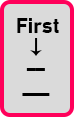In the beginning pointer ‘first’ is null. There is nothing. So, we want to insert 1st node. We will write ‘Insert (0, 8)’. Here the first parameter is index and next is the data we want to insert. Here we can insert at only 0th position as there are no nodes.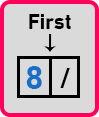Here we have inserted first node. Now here we have two options for insertion. We can insert before first node or after the first node. Let us insert after first node, so we will write ‘Insert (1, 3)’.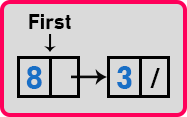So new node ‘3’ is inserted after first node. Now we have 2 nodes so we have 3 places to insert a new node. First is before the 1st node, second between the two nodes and third is after the last node. Let us insert after last node so we will write ‘Insert (2, 6)’.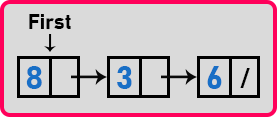New node ‘6’ is inserted after the last node. Now let us insert at before the first node, so we will write ‘Insert (0, 4)’. As we are inserting before the first node so we have to modify the first pointer then point it to the new node which we have inserted.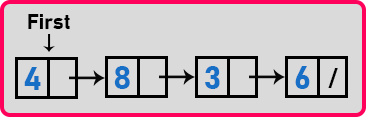Now we will insert after 3rd index which is after ‘3’ node. So, we will write ‘Insert (3, 7)’.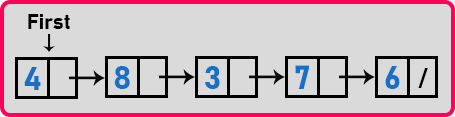So, by using the insert function we can create a linked list. We have to remember when we create the first node its indices must be zero and after creating the first node, we must give valid indices to create nodes. Once we have created a linked list then we can perform all other operations on the link list like counting the nodes, adding all the elements, searching or reversing etc. all these operations we can do.

##### Program to create a linked list using Insert in C Language:
```#include <stdio.h>
#include <stdlib.h>

struct Node
{
int data;
struct Node *next;
} *first = NULL;

void Create(int A[], int n)
{
int i;
struct Node *t, *last;
first = (struct Node *) malloc(sizeof (struct Node));
first->data = A;
first->next = NULL;
last = first;

for (i = 1; i < n; i++)
{
t = (struct Node *) malloc(sizeof (struct Node));
t->data = A[i];
t->next = NULL;
last->next = t;
last = t;
}
}

void Display(struct Node *p)
{
while (p != NULL)
{
printf ("%d ", p->data);
p = p->next;
}
}

int Count(struct Node *p)
{
int l = 0;
while (p)
{
l++;
p = p->next;
}
return l;
}

void Insert(struct Node *p, int index, int x)
{
struct Node *t;
int i;

if (index < 0 || index > Count(p))
return;
t = (struct Node *) malloc (sizeof (struct Node));
t->data = x;

if (index == 0)
{
t->next = first;
first = t;
}
else
{
for (i = 0; i < index - 1; i++)
p = p->next;
t->next = p->next;
p->next = t;
}
}

int main()
{
Insert (first, 0, 6);
Insert (first, 0, 7);
Insert (first, 0, 3);
Insert (first, 0, 4);
Insert (first, 1, 8);
Display (first);
return 0;
}
```
###### Output: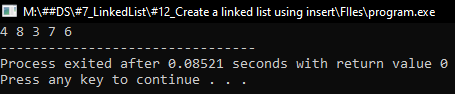##### Creating a Linked List by Inserting at Last:

Now, we will see a method in which we will create a linked list by inserting nodes at last position.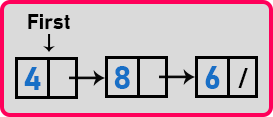Here we have linked list. In this linked list, we can insert at any position but we will insert at the end of the linked list. Suppose we want to insert ‘7’ then it will be inserted as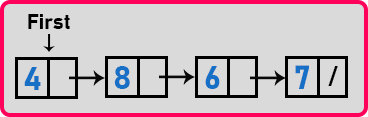Here ‘7’ is inserted at the end of the linked list. We know very well that for inserting a new node at the last, we should take a pointer and point that to the node after which we want to insert a node. But when we are repeatedly inserting at the last only then one solution is we can maintain one pointer ‘last’ on the last node, that will help us in inserting.

Suppose we want to insert a new value that is ‘5’. So, we will create a new node by using a temporary pointer and insert the value. And point the ‘last’ to the pointer to this node.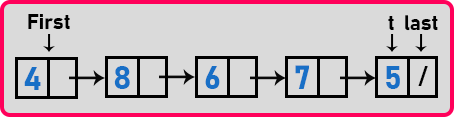So, with the help of the ‘last’ pointer, we can insert it at the end of a linked list in just a constant time because we don’t have to traverse the linked list. Insertion in constant time is possible if we are always inserting at the last. Then let us show it from the beginning. Suppose I have just one node ‘8’ then ‘first’ will point on this node and ‘last’ should also be on this node as there are no nodes.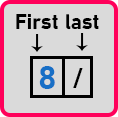So, if there is only one node then bring ‘first’ and ‘last’ point on that same node. This is a special case that we have to handle. Now let us add more on this. Create a new node with a temporary pointer ‘t’ and insert the value ‘4’ and point ‘last’ on it.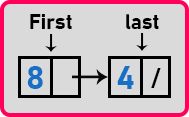Now one more case, if suppose there are no nodes. Then both the ‘first’ and ‘last’ should be null.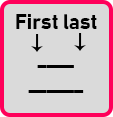When there are no nodes initially then both the pointers must be null. An empty linked list when we insert a new node then ‘first’ and ‘last’ both will point on that node.

Now let us write a function for insertion at last in linked list.

```void InsertLast(int x)
{
struct Node *t;
t = (struct Node *) malloc (sizeof (struct Node));
t->data = x;
t->next = NULL;
if (first == NULL)
{
first = last = t;
}
else
{
last->next = t;
last = t;
}
}
```

Now let us write program for insertion at last in linked list.

##### Program for insertion at last in linked list using C Language:
```#include <stdio.h>
#include <stdlib.h>

struct Node
{
int data;
struct Node *next;
}   *first = NULL, *last = NULL;

void Create(int A[], int n)
{
int i;
struct Node *t, *last;
first = (struct Node *) malloc(sizeof (struct Node));
first->data = A;
first->next = NULL;
last = first;

for (i = 1; i < n; i++)
{
t = (struct Node *) malloc(sizeof (struct Node));
t->data = A[i];
t->next = NULL;
last->next = t;
last = t;
}
}

void Display (struct Node *p)
{
while(p != NULL)
{
printf ("%d ", p->data);
p = p->next;
}
}

void InsertLast(int x)
{
struct Node *t;
t = (struct Node *) malloc (sizeof (struct Node));
t->data = x;
t->next = NULL;
if (first == NULL)
{
first = last = t;
}
else
{
last->next = t;
last = t;
}
}

int main()
{
InsertLast (4);
InsertLast (8);
InsertLast (6);
InsertLast (7);
Display (first);
return 0;
}
```
###### Output: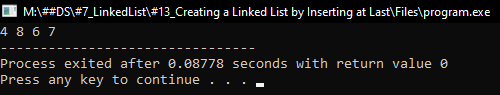In the next article, I am going to discuss Inserting in a Sorted Linked List with Examples. Here, in this article, I try to explain Create a Linked list using Insert using C Language with Examples and I hope you enjoy this Create a Linked list using Insert using C Language with Examples article.